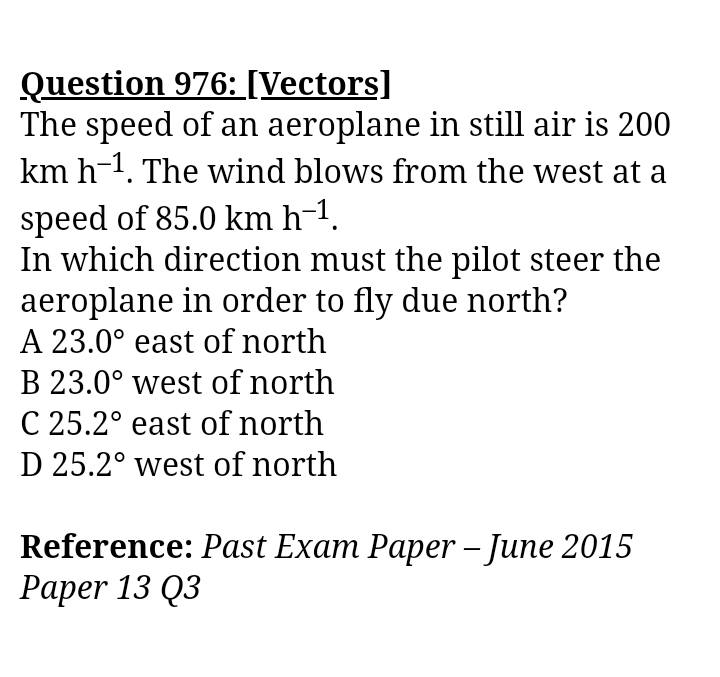# Hard time with this Vector Velocity Problem

• KevMilan

#### KevMilan

Summary: I've posted this in a few forums but still confused on this problem. If the plane is moving at 200km/h and the wind pushes the plane with a velocity of 85km/h, then the resultant velocity would be 217km/h, and using sine inverse, 217.sin(theta=85, I got 23°, which is B, but the answer key shows D.[Moderator's note: Moved from a technical forum and thus no template.]

Last edited by a moderator:

Where did you get the 217km/h?

Did you draw a diagram?

Where did you get the 217km/h?

Did you draw a diagram?
I used pythagoras theorem, I assumed 200km/h as facing north and 85km/h facing east, and the hypotenuse would be 217km/h.

The hypotenuse should be the 200 km/h in this problem

I used pythagoras theorem, I assumed 200km/h as facing north and 85km/h facing east, and the hypotenuse would be 217km/h.

But then the 217km/h is not is a northerly direction (relative to the ground). It is north relative to the air. That's what would happen if you tried to fly north, but didn't take into account the air which is then blowing you eastwards.

The hypotenuse should be the 200 km/h in this problem
A lot of sites have been saying this but I still don't get why the hypotenuse is 200, can you kindly explain this in detail?

A lot of sites have been saying this but I still don't get why the hypotenuse is 200, can you kindly explain this in detail?

Draw a diagram with the paper being the ground. You want to fly north relative to the ground. You might like to draw the air velocity vector first. Then, you should see the direction of the "plane relative to air" vector that is needed to result in a northerly path.

With your approach you are defintely going east. Imagine you were trying to walk across a room and I was pushing you sideways. If you try to walk straight, then you'll end up off course. In order to move directly across the room, you would have to walk into me slightly. In this case, and in the case of your problem, the external force is slowing you down, because you are moving into it. That's why the ground speed will be less than 200km/h.

Let ##\vec{e}_x## point from west to east and ##\vec{e}_y## from south to north. Then
$$\vec{v}=v_{\text{wind}} \vec{e}_x + \vec{v}_{\text{plane}}.$$
You want the plane going towards north, i.e.,
$$\vec{e}_x \cdot \vec{v}=0 \; \Rightarrow \; v_{\text{plane} x=-v_{\text{wind}}.$$
Now you only need ##|\vec{v}_{\text{plane}}|=200 \;\text{km}/{\text{h}}## to get ##v_{\text{plane}y}##, and from this result you also get the angle.

A lot of sites have been saying this but I still don't get why the hypotenuse is 200, can you kindly explain this in detail?
You have three velocities:

The plane wrt the air (direction unknown, magnitude 200 kph)
The air wrt the ground (direction west, magnitude 85 kph)
The plane wrt the ground (direction north, magnitude unknown)

The last two form a right angle (north and west) so the first must be the hypotenuse.

You have three velocities:

The plane wrt the air (direction unknown, magnitude 200 kph)
The air wrt the ground (direction west, magnitude 85 kph)
The plane wrt the ground (direction north, magnitude unknown)

The last two form a right angle (north and west) so the first must be the hypotenuse.
When you say with respect to air, basically whatever the wind speed is, it'll always be moving at 200km/h in the wind's perspective, right? So the word 'still air' in the question is crucial to finding this solution?

Yes. It is also called “air speed”

Another way to describe what needs to be done: The plane needs a heading such that its East-West component of its 200 km/hr cancels the wind's 85 km/hr.

•vanhees71 and PeroK
That's what I've said in #8. All you have to do is to put the numbers in. It also may help a lot to draw the vectors to immediately see, how it works out geometrically (it's simple trigonometry of a right triangle).